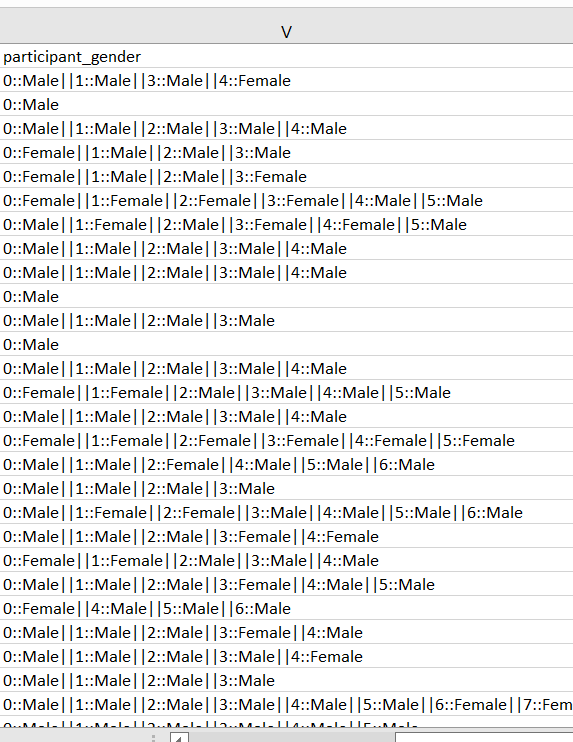# WORD FREEQUENCIES PROBLEM

Member Posts: 2Contributor I
Hi, anyone know how can I calculate the frequencies of each males and females? I want to create two new column which are female and male with its frequencies for each rows.• Administrator, Moderator, Employee, RapidMiner Certified Analyst, RapidMiner Certified Expert Posts: 901Unicorn
Hi!

This is a nice challenge. It can work with a combination of Split, De-Pivot, Split, Aggregate and Pivot.

First you should check your import process. participant_gender should be the attribute name, not the first data entry.

Do you have an ID elsewhere in your data? If not, you can use Generate ID to identify the rows.

Then use Split with the || separator. Split uses regular expression syntax, so it will be \|\| for this separator value. This will create a number of additional columns with single X::Male or Y::Female entries.

You can then use De-Pivot to put these columns into the rows based on the ID. You will get multiple entries for every ID.

This can again be split up with Split on the separator ::. This gives you the genders in an attribute. You can then use Aggregate to group on the ID and the gender and count the numbers. If you need these on new attributes, use Pivot to transfer the gender values on the columns.

Regards,
Balázs
• Administrator, Employee, RapidMiner Certified Analyst, Member Posts: 266Unicorn
Hey @NewbieStudent

You can use this approach.

```<?xml version="1.0" encoding="UTF-8"?><process version="9.10.011">
<context>
<input/>
<output/>
<macros/>
</context>
<operator activated="true" class="process" compatibility="9.10.011" expanded="true" name="Process">
<parameter key="logverbosity" value="init"/>
<parameter key="random_seed" value="-1"/>
<parameter key="send_mail" value="never"/>
<parameter key="process_duration_for_mail" value="30"/>
<parameter key="encoding" value="UTF-8"/>
<process expanded="true">
<operator activated="true" class="utility:create_exampleset" compatibility="9.10.011" expanded="true" height="68" name="Create ExampleSet" width="90" x="45" y="34">
<parameter key="generator_type" value="comma separated text"/>
<parameter key="number_of_examples" value="100"/>
<parameter key="use_stepsize" value="false"/>
<list key="function_descriptions"/>
<list key="numeric_series_configuration"/>
<list key="date_series_configuration"/>
<list key="date_series_configuration (interval)"/>
<parameter key="date_format" value="yyyy-MM-dd HH:mm:ss"/>
<parameter key="time_zone" value="SYSTEM"/>
<parameter key="input_csv_text" value="VALUES&#10;0::Male||1:Male||3:Male||4::Female&#10;0::Male||1:Female||3:Male||4::Female&#10;0::Male||1:Male"/>
<parameter key="column_separator" value=","/>
<parameter key="parse_all_as_nominal" value="false"/>
<parameter key="decimal_point_character" value="."/>
<parameter key="trim_attribute_names" value="true"/>
</operator>
<operator activated="true" class="nominal_to_text" compatibility="9.10.011" expanded="true" height="82" name="Nominal to Text" width="90" x="179" y="34">
<parameter key="attribute_filter_type" value="single"/>
<parameter key="attribute" value="VALUES"/>
<parameter key="attributes" value=""/>
<parameter key="use_except_expression" value="false"/>
<parameter key="value_type" value="nominal"/>
<parameter key="use_value_type_exception" value="false"/>
<parameter key="except_value_type" value="file_path"/>
<parameter key="block_type" value="single_value"/>
<parameter key="use_block_type_exception" value="false"/>
<parameter key="except_block_type" value="single_value"/>
<parameter key="invert_selection" value="false"/>
<parameter key="include_special_attributes" value="false"/>
</operator>
<operator activated="true" class="text:process_document_from_data" compatibility="9.4.000" expanded="true" height="82" name="Process Documents from Data" width="90" x="313" y="34">
<parameter key="create_word_vector" value="true"/>
<parameter key="vector_creation" value="Term Occurrences"/>
<parameter key="keep_text" value="false"/>
<parameter key="prune_method" value="none"/>
<parameter key="prune_below_percent" value="3.0"/>
<parameter key="prune_above_percent" value="30.0"/>
<parameter key="prune_below_rank" value="0.05"/>
<parameter key="prune_above_rank" value="0.95"/>
<parameter key="datamanagement" value="double_sparse_array"/>
<parameter key="data_management" value="auto"/>
<parameter key="select_attributes_and_weights" value="false"/>
<list key="specify_weights"/>
<process expanded="true">
<operator activated="true" class="text:transform_cases" compatibility="9.4.000" expanded="true" height="68" name="Transform Cases" width="90" x="112" y="34">
<parameter key="transform_to" value="lower case"/>
</operator>
<operator activated="true" class="text:tokenize" compatibility="9.4.000" expanded="true" height="68" name="Tokenize" width="90" x="246" y="34">
<parameter key="mode" value="non letters"/>
<parameter key="characters" value=".:"/>
<parameter key="language" value="English"/>
<parameter key="max_token_length" value="3"/>
</operator>
<connect from_port="document" to_op="Transform Cases" to_port="document"/>
<connect from_op="Transform Cases" from_port="document" to_op="Tokenize" to_port="document"/>
<connect from_op="Tokenize" from_port="document" to_port="document 1"/>
<portSpacing port="source_document" spacing="0"/>
<portSpacing port="sink_document 1" spacing="0"/>
<portSpacing port="sink_document 2" spacing="0"/>
</process>
</operator>
<connect from_op="Create ExampleSet" from_port="output" to_op="Nominal to Text" to_port="example set input"/>
<connect from_op="Nominal to Text" from_port="example set output" to_op="Process Documents from Data" to_port="example set"/>
<connect from_op="Process Documents from Data" from_port="example set" to_port="result 1"/>
<portSpacing port="source_input 1" spacing="0"/>
<portSpacing port="sink_result 1" spacing="0"/>
<portSpacing port="sink_result 2" spacing="0"/>
</process>
</operator>
</process>```